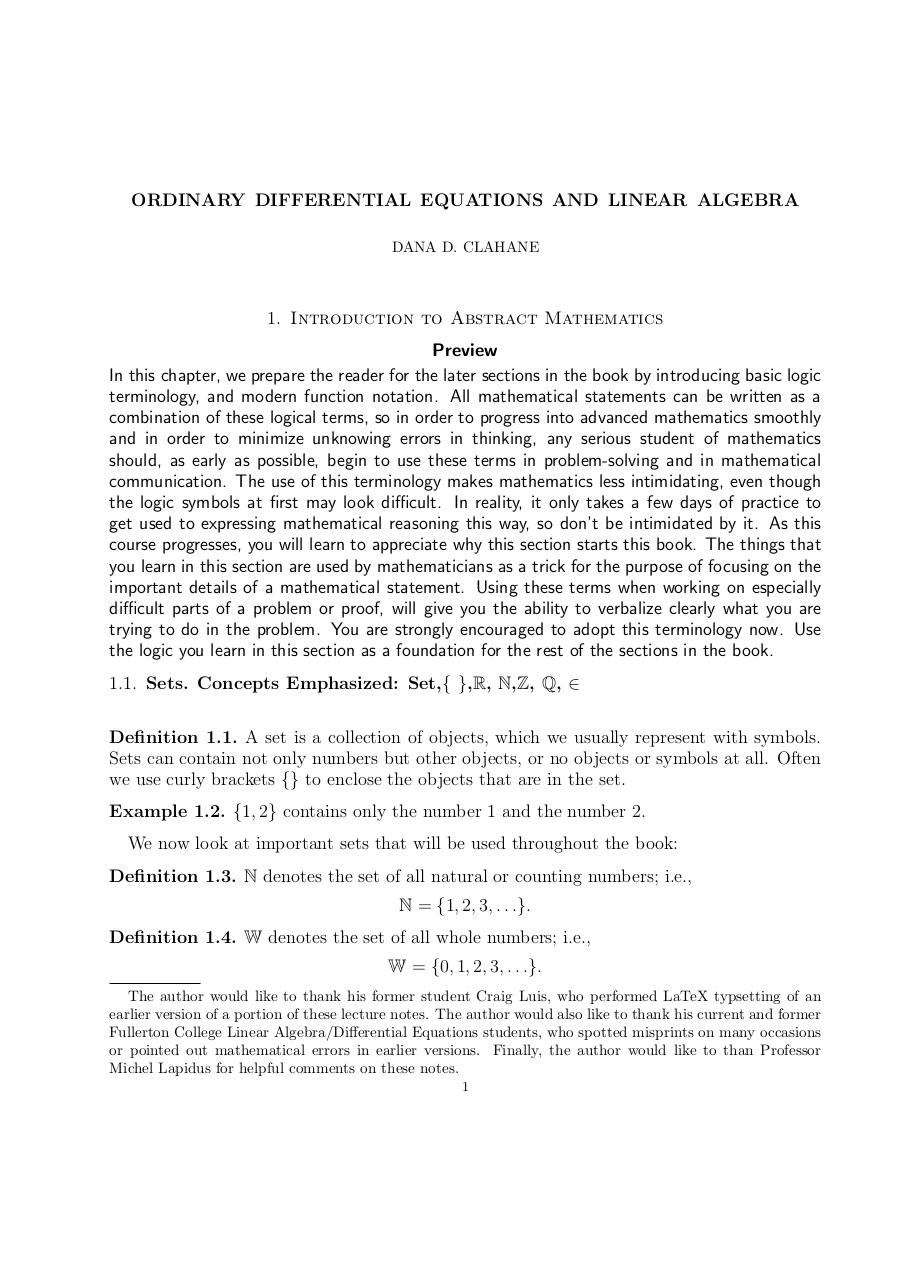# ODELAClahane061416.pdfPage 12370

#### Text preview

ORDINARY DIFFERENTIAL EQUATIONS AND LINEAR ALGEBRA
DANA D. CLAHANE

1. Introduction to Abstract Mathematics
Preview
In this chapter, we prepare the reader for the later sections in the book by introducing basic logic
terminology, and modern function notation. All mathematical statements can be written as a
combination of these logical terms, so in order to progress into advanced mathematics smoothly
and in order to minimize unknowing errors in thinking, any serious student of mathematics
should, as early as possible, begin to use these terms in problem-solving and in mathematical
communication. The use of this terminology makes mathematics less intimidating, even though
the logic symbols at first may look difficult. In reality, it only takes a few days of practice to
get used to expressing mathematical reasoning this way, so don’t be intimidated by it. As this
course progresses, you will learn to appreciate why this section starts this book. The things that
you learn in this section are used by mathematicians as a trick for the purpose of focusing on the
important details of a mathematical statement. Using these terms when working on especially
difficult parts of a problem or proof, will give you the ability to verbalize clearly what you are
trying to do in the problem. You are strongly encouraged to adopt this terminology now. Use
the logic you learn in this section as a foundation for the rest of the sections in the book.
1.1. Sets. Concepts Emphasized: Set,{ },R, N,Z, Q, ∈
Definition 1.1. A set is a collection of objects, which we usually represent with symbols.
Sets can contain not only numbers but other objects, or no objects or symbols at all. Often
we use curly brackets {} to enclose the objects that are in the set.
Example 1.2. {1, 2} contains only the number 1 and the number 2.
We now look at important sets that will be used throughout the book:
Definition 1.3. N denotes the set of all natural or counting numbers; i.e.,
N = {1, 2, 3, . . .}.
Definition 1.4. W denotes the set of all whole numbers; i.e.,
W = {0, 1, 2, 3, . . .}.
The author would like to thank his former student Craig Luis, who performed LaTeX typsetting of an
earlier version of a portion of these lecture notes. The author would also like to thank his current and former
Fullerton College Linear Algebra/Differential Equations students, who spotted misprints on many occasions
or pointed out mathematical errors in earlier versions. Finally, the author would like to than Professor